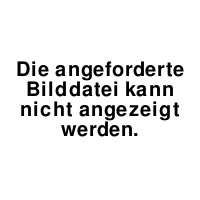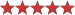## Carry the Three (two-digit arrow sudoku)

(Eingestellt am 30. Oktober 2020, 18:10 Uhr von clover)

Normal sudoku rules apply.

Digits along an arrow sum to the value in the corresponding circle. When the circle contains two digits, read them as a single two-digit number from left to right.

The two digits separated by a white dot differ by exactly 1.

f-puzzles

If you aren't making headway on this puzzle, I've included an explanation of the breakin in white text below the image. Highlight it to read! :)SEQUENTIAL HINTS FOR BREAK-IN (highlight text below):

1.

Highlight all of the squares associated with the four circles on the top row. Notice that these squares make up three complete 3x3 blocks, plus four additional squares in columns 8 and 9.

2.

What is the sum of your highlighted squares from the previous step? The sum of the three full 3x3 blocks is 45+45+45=135. There's a 3 already given, and the other three "extra" squares are all in the same 3x3 block, meaning that their sum is at most 7+8+9=24. So, the largest possible sum of your highlighted squares is 135+3+24 = 162. Now, what's the smallest possible sum?

3.

Now, try making the values along the arrows in the top three blocks as small as you can. To do this, you need to use the smallest possible digits for the TENS digits of the two-digit numbers in the circles: 1, 2, 3, and 4 would be the tens digits. To make the overall values as small as possible, use 5, 6, 7, and 8 (in some order) for the UNITS digits. 9 is left over.

In this scenario, the minimum sum of your highlighted squares is 10 + 20 + 30 + 40 (using 1 through 4 as tens digits) plus 5 + 6 + 7 + 8 (ones digits), plus 1+2+3+4+5+6+7+8 (because the digits in the circles themselves aren't included in the arrow sums, so we also need to add those digits in.) That total is 100 + 26 + 36, or 162.

4.

That's the key to the break-in! The smallest possible value of those highlighted squares is 162, and the largest possible value is also 162. Therefore, the ONLY possible value is 162. So, you know the tens digits on the top row are 1, 2, 3, and 4 (in some order) and the units digits are 5, 6, 7, and 8 (in some order). Any different arrangement would make the sum larger than the maximum. (You can also conclude that the "extra" squares in box 6 are 7, 8, and 9.)

If you get stuck again after this point:

Apply the exact same technique to the second group of four 2-digit arrow sums, noticing that the unknown digits in row 7 sum to 45-3 = 42.

Lösungscode: row 6

Zuletzt geändert am 31. Oktober 2020, 11:45 Uhr

Gelöst von ThrowngNinja, primovera, smckinley, MeloMania!, kelkoo97, NikolaZ, marcmees, dandbdi, SirWoezel, Gliperal, Ours brun, zorant, Mody, polar, Ragna
Komplette Liste

### Kommentare

am 9. November 2020, 13:36 Uhr von marcmees
didn't need the hints. very hard but rewarding solving path.

am 31. Oktober 2020, 11:45 Uhr von clover
Added extensive (white text) hints for the breakin!

am 31. Oktober 2020, 11:29 Uhr von clover
increased the difficulty rating given that only one person has solved it so far! :o

 Schwierigkeit:Bewertung: 100 % Gelöst: 15 mal Beobachtet: 4 mal ID: 0004ME

Lösungscode:

## Anmelden